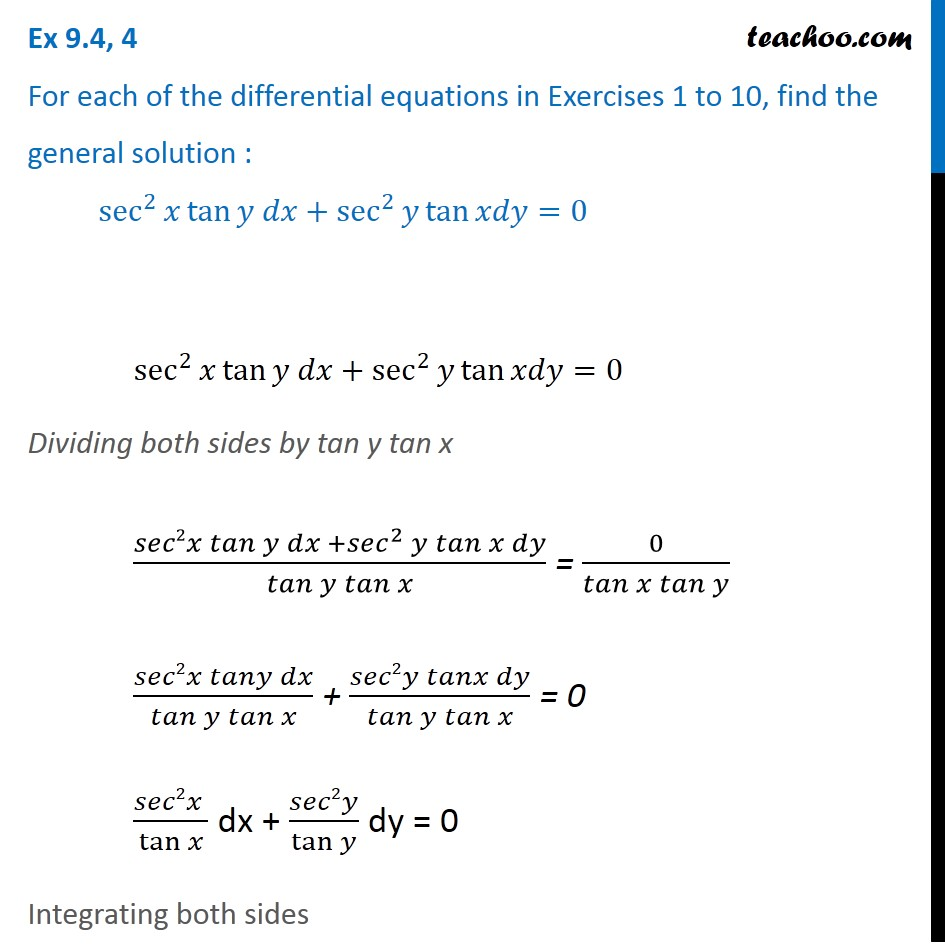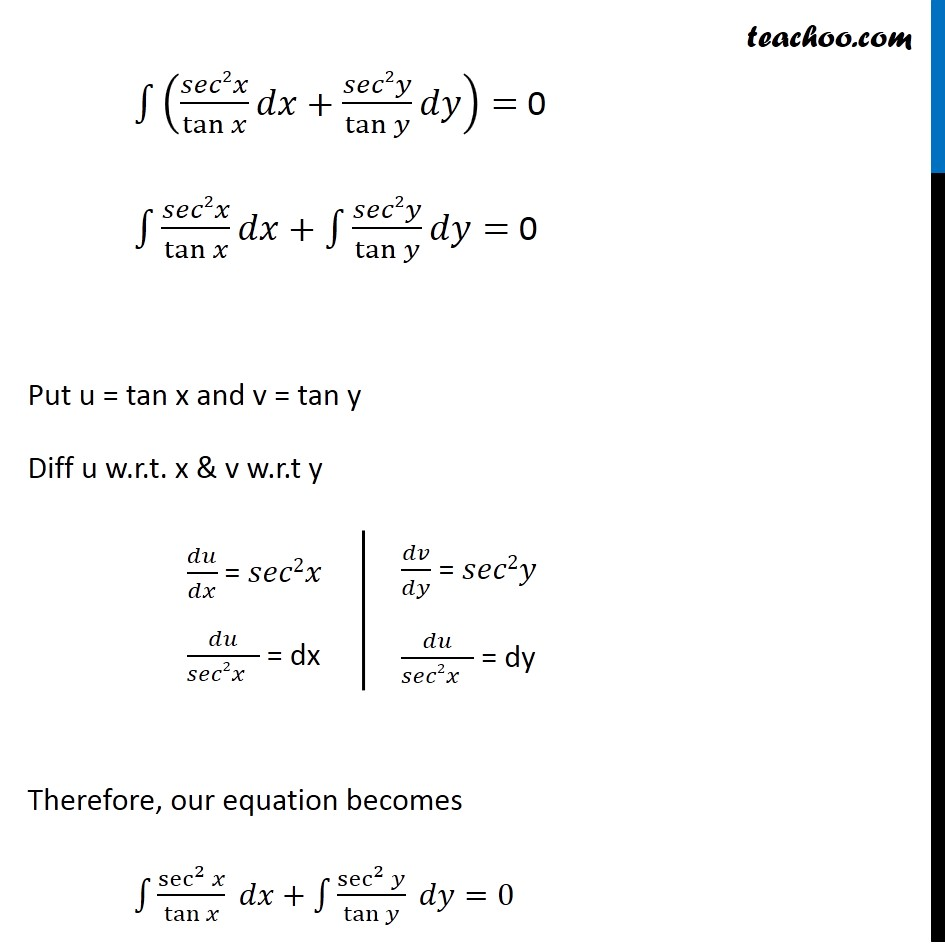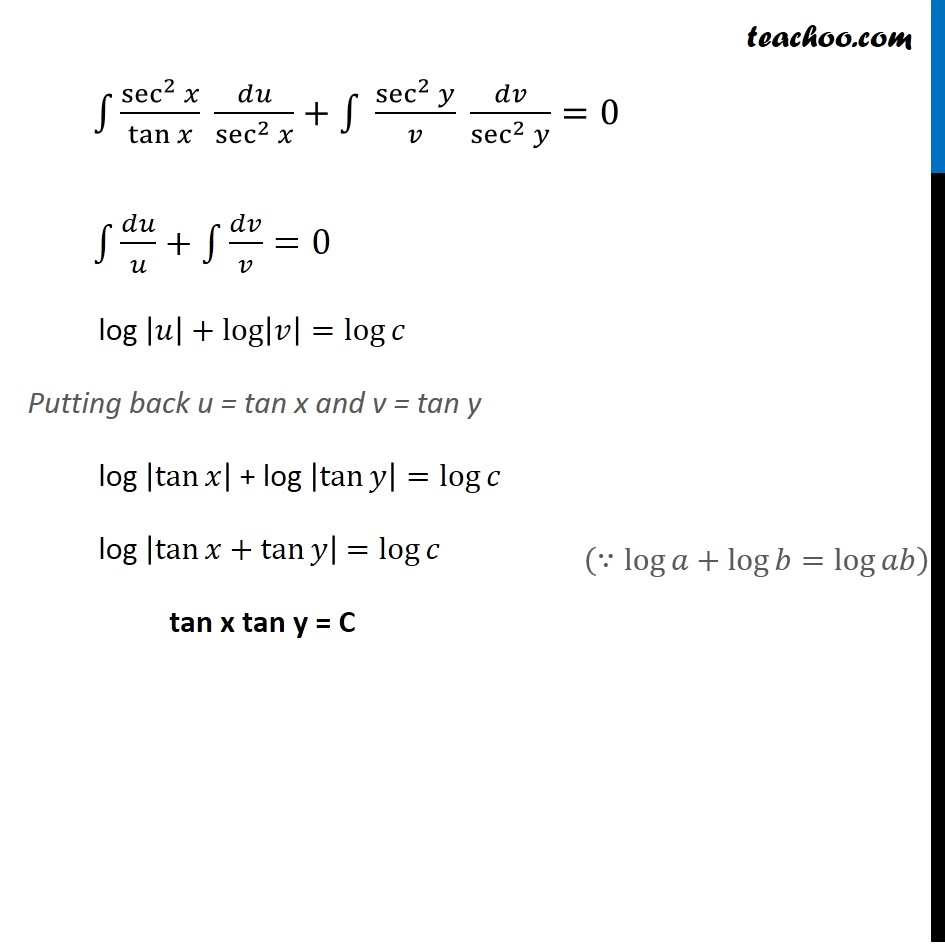Ex 9.3

Chapter 9 Class 12 Differential Equations
Serial order wiseLearn in your speed, with individual attention - Teachoo Maths 1-on-1 Class

### Transcript

Ex 9.3, 4 For each of the differential equations in Exercises 1 to 10, find the general solution : sec^2⁡〖𝑥 tan⁡〖𝑦 𝑑𝑥+sec^2⁡〖𝑦 tan⁡〖𝑥𝑑𝑦=0〗 〗 〗 〗 sec^2⁡〖𝑥 tan⁡〖𝑦 𝑑𝑥+sec^2⁡〖𝑦 tan⁡〖𝑥𝑑𝑦=0〗 〗 〗 〗 Dividing both sides by tan y tan x (𝑠𝑒𝑐2𝑥 𝑡𝑎𝑛⁡𝑦 𝑑𝑥 +〖𝑠𝑒𝑐〗^2⁡𝑦 𝑡𝑎𝑛⁡〖𝑥 𝑑𝑦〗)/𝑡𝑎𝑛⁡〖𝑦 𝑡𝑎𝑛⁡𝑥 〗 = 0/𝑡𝑎𝑛⁡〖𝑥 𝑡𝑎𝑛⁡𝑦 〗 (𝑠𝑒𝑐2𝑥 𝑡𝑎𝑛𝑦 𝑑𝑥)/𝑡𝑎𝑛⁡〖𝑦 𝑡𝑎𝑛⁡𝑥 〗 + (𝑠𝑒𝑐2𝑦 𝑡𝑎𝑛𝑥 𝑑𝑦)/𝑡𝑎𝑛⁡〖𝑦 𝑡𝑎𝑛⁡𝑥 〗 = 0 (𝑠𝑒𝑐2𝑥 )/tan⁡𝑥 dx + 𝑠𝑒𝑐2𝑦/tan⁡𝑦 dy = 0 Integrating both sides ∫1▒〖(𝑠𝑒𝑐2𝑥/tan⁡𝑥 𝑑𝑥+𝑠𝑒𝑐2𝑦/tan⁡𝑦 𝑑𝑦)=〗 0 ∫1▒〖𝑠𝑒𝑐2𝑥/tan⁡𝑥 𝑑𝑥+∫1▒〖𝑠𝑒𝑐2𝑦/tan⁡𝑦 𝑑𝑦〗=〗 0 Put u = tan x and v = tan y Diff u w.r.t. x & v w.r.t y 𝑑𝑢/𝑑𝑥 = 𝑠𝑒𝑐2𝑥 𝑑𝑢/𝑠𝑒𝑐2𝑥" " = dx 𝑑𝑣/𝑑𝑦 = 𝑠𝑒𝑐2𝑦 𝑑𝑢/𝑠𝑒𝑐2𝑥" " = dy Therefore, our equation becomes ∫1▒〖sec^2⁡𝑥/tan⁡𝑥 𝑑𝑥〗+∫1▒〖sec^2⁡𝑦/tan⁡𝑦 𝑑𝑦=0〗 ∫1▒〖sec^2⁡𝑥/tan⁡𝑥 𝑑𝑢/sec^2⁡𝑥 〗+∫1▒〖 sec^2⁡𝑦/𝑣 𝑑𝑣/sec^2⁡𝑦 =0〗 ∫1▒〖𝑑𝑢/𝑢+∫1▒〖𝑑𝑣/𝑣=0〗〗 log |𝑢|+log⁡|𝑣|=log⁡𝑐 Putting back u = tan x and v = tan y log |tan⁡𝑥 | + log |tan⁡𝑦 |=log⁡𝑐 log |tan⁡〖𝑥+tan⁡𝑦 〗 | =log⁡𝑐 tan x tan y = C (∵log⁡〖𝑎+log⁡〖𝑏=log⁡𝑎𝑏 〗 〗 )